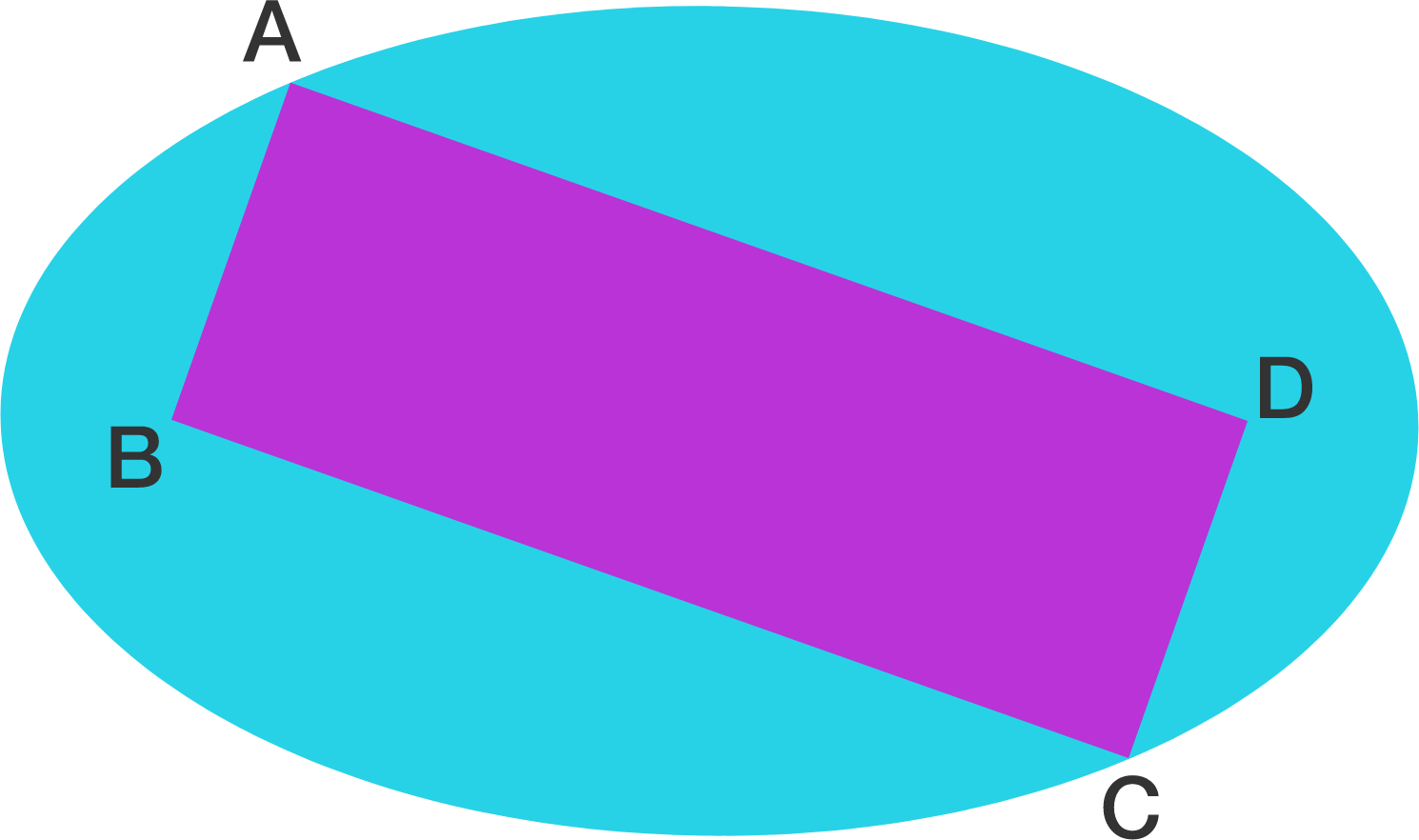Rectangle & Ellipse

Geometry Level 5The picture above shows a rectangle ABCD with area $200$ inside an ellipse with area $200 \pi$ passes through vertex $A$ and $C$, and has foci at $B$ and $D$.

Find the perimeter of the rectangle.

×